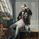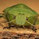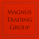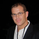11349 görüntülenme
11349
Guppy Multiple Moving Averages
---------------------------------
Developed by Daryl Guppy, the basic idea of Multiple moving average( MMA ) is to view the trend as two band of moving averages – short term band and long term band.

Shortterm averages capture the inferred behaviour of traders and long term represents the investors. Uses fractal repetition to identify points of agreement and disagreement which precede significant trend changes.

Short intro on interpreting the signals:

Guppy Oscillator
---------------------------------
The Guppy MMA Oscillator, developed by Leon Wilson, is an oscillator representation of difference between GMMA ribbons. Look for signal crosses for the triggers.

Linda Raschke (3/10) Oscillator
---------------------------------
This oscillator is similar to having a MACD of (3,10,16), the nuances are explained by Linda Raschke in her manual "Professional Trading Techniques":
http://www.lbrgroup.com/images//Linda%27...

Ian Oscillator
---------------------------------
Simple EMA difference converted to an oscillator. Use the signal crosses as triggers.
```//
// @author LazyBear
//
// If you use this code in its original/modified form, do drop me a note.
//
study("Guppy MMA [LazyBear]", shorttitle="GMMA_LB", overlay=true)

src=close

// shortterm
stsl=plot(ema(src, 3), color=#000066, linewidth=1)
plot(ema(src, 5), color=#000099)
plot(ema(src, 8), color=#0000cc)
plot(ema(src, 10), color=#0000ff)
plot(ema(src, 12), color=#0033cc)
stll=plot(ema(src, 15), color=#0033ff)

// longterm
ltsl=plot(ema(src, 30), color=#990000, linewidth=1)
plot(ema(src, 35), color=#990033)
plot(ema(src, 40), color=#cc0000)
plot(ema(src, 45), color=#cc0033)
plot(ema(src, 50), color=#ff0000)
ltll=plot(ema(src, 60), color=#ff0033)

fill(stsl, stll, blue)
fill(ltsl, ltll, red)```Hi, great work! I am very interested in the three oscillators, where can I find the script to add them to my charts? thank you very much
Cevap Gönderwa1
I found the code - but it seems to be in AFL. I am new to TV and still learning how the code works.

Can someone make this work with TV?

Trigger = Param("Trigger",21,1,55,1);

Short1=(EMA(Close,3)+EMA(Close,5)+
EMA(Close,8)+EMA(Close,10)+
EMA(Close,12)+EMA(Close,15));

LONG2 =(EMA(Close,30)+EMA(Close,35)+
EMA(Close,40)+EMA(Close,45)+
EMA(Close,50)+EMA(Close,60));

GuppyMMAOscillator = ((Short1-Long2)/Long2)*100;

Plot (GuppyMMAOscillator, "Guppy MMA Oscillator", colorRed);

Plot (EMA(GuppyMMAOscillator, Trigger), "Trigger", colorBrightGreen);

Plot (0, "", colorWhite, styleNoLabel);
Cevap Gönderand again: thx for you work :-)

And one other method to use MMA or WMA for finding the tops is using the divergence of them in multible timefame. As more Divergence in multilble timeframe as more the chance that it will be the top.
Cevap Gönder